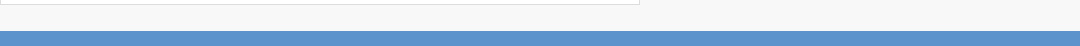# ROI: AN INVESTMENT CONSIDERATION

ROI stands for Return on Investment, which is a financial metric used to evaluate the profitability of an investment relative to its cost.It is a commonly used metric in business and finance to determine whether an investment is worthwhile and to compare the performance of different investment options.

ROI is calculated by dividing the net profit (the return) of an investment by the cost of the investment, and expressing the result as a percentage.The net profit is the amount earned from the investment minus the cost of the investment.The cost of the investment can include any expenses related to the investment, such as the purchase price of an asset, transaction fees, and ongoing maintenance costs.

The formula for calculating ROI is as follows:

ROI = (Net Profit / Cost of Investment) x 100%

For example, if you invest \$10,000 in a business venture and earn a net profit of \$15,000, your ROI would be calculated as follows:

ROI = (\$15,000 / \$10,000) x 100% = 150%

This means that your investment generated a return of 150%, or \$15,000 on your original investment of \$10,000.

However, ROI has some limitations.It does not take into account the time value of money, which means that it does not consider the fact that money invested today is worth more than the same amount of money invested in the future.It also does not account for risk, which means that an investment with a higher ROI may be riskier than an investment with a lower ROI.

Therefore, it is important to consider other facts, in addition to ROI, such as the potential risks, the time horizon of the investment, market conditions, and future growth when evaluating an investment opportunity.

Categories: General